### Add Up (Sum) Entire Columns or Rows in Excel

This tutorial covers how to add entire rows or columns in Excel.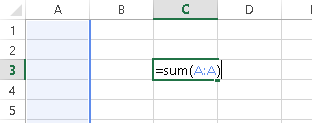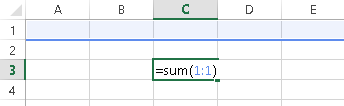## The Sum Function

We will use the Sum Function to add up entire rows and columns.

`= SUM(number1, number2, …)`

The Sum Function takes input in two primary forms: standalone cell references (ex.=sum(a1,b2,c3)) or an array of cells (=sum(A1:E1)). We will use the latter method: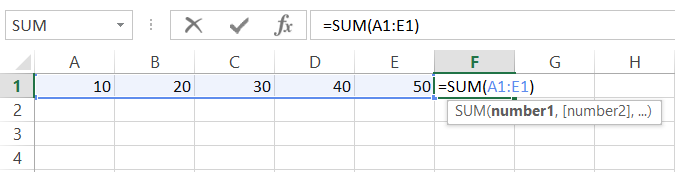Tip 1: The Shortcut ALT + = (press and hold ALT then tap =) will automatically create a Sum Function. When possible, Excel will guess which cells you would like to sum together, populating the Sum Function.
Tip 2: After using the ALT + = shortcut or after typing =sum(, use the arrow keys to select the appropriate cell. Then hold down SHIFT or CTRL + SHIFT to select the desired range of cells.
Tip 3: Instead of using the keyboard, you can also use the mouse to drag and highlight the desired range and complete the formula.

## Sum an Entire Column

To add up an entire column, enter the Sum Function: =sum( and then select the desired column either by clicking the column letter at the top of the screen or by using the arrow keys to navigate to the column and using the CTRL + SPACE shortcut to select the entire column. The formula will be in the form of =sum(A:A).## Sum an Entire Row

To add up an entire column, enter the Sum Function: =sum( and then select the row either by clicking the row number on the left of the screen or by navigating to the desired row with the arrow keys and using the SHIFT + SPACE shortcut to select the entire row. The formula will be in the form of =sum(1:1).## Add up Multiple Columns or Rows at Once

To sum columns or rows at the same time, use a formula of the form: =sum(A:B) or =sum(1:2). Remember that you can also use the keyboard shortcuts CTRL + SPACE to select an entire column or SHIFT + SPACE an entire row. Then, while holding down SHIFT, use the arrow keys to select multiple rows.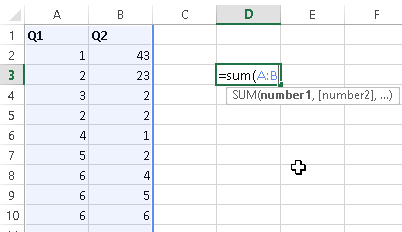## Sum Non-Contiguous Columns or Rows at Once

There are several ways to add up non-contiguous rows or columns at once. Of course, you can create multiple Sum Formulas and add the results together. However, you can also use a comma delimiter within the Sum Function to enter multiple arrays of numbers within the same Sum Function.

Note the difference,
Multiple Sum Functions: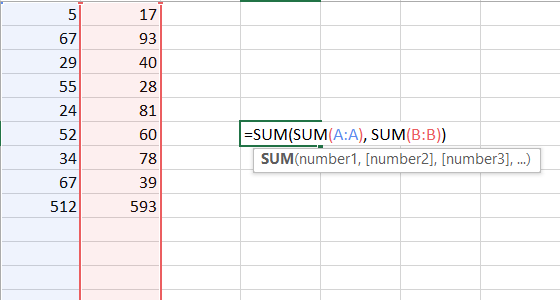Comma Delimiter: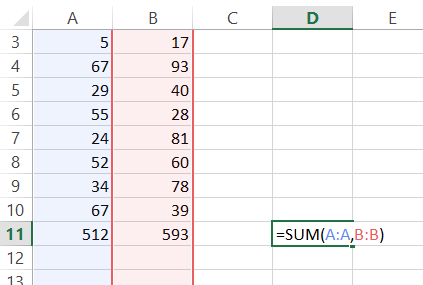## Sum Everything Except the Header

Often times, you need to add up an entire column (or row), except the header. In Excel 2013, non-integer entries included in the Sum formula are automatically ignored. If you included cell A1 (the header) in the Sum Function: =sum(A:A), the Sum Function would work properly. However, it is good practice to maintain backward compatibility.

Instead, you can use formula =SUM(A2:A1048576). Why 1,048,576? Excel worksheets only have 1,048,576 rows!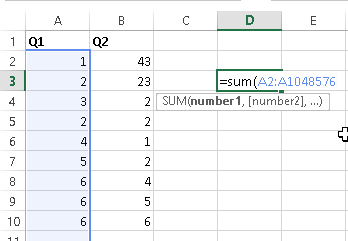To see this, select a cell in a blank column. Then you use the shortcut: CTRL + Down Arrow to navigate to the last row in the worksheet.

## Add Up all Rows Containing Data

Instead of adding up an entire column to the bottom of the worksheet, you can add-up only the rows containing data. To do this, first start your SUM Function. Then select the first row in the column containing the data you wish to sum, then use CTRL + SHIFT + Down Arrow to select all the cells in that column (Note: be careful of blank cells. CTRL + SHIFT + Arrow will navigate to the cell directly before a blank cell)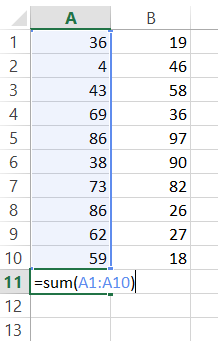## Autosum Magic

Select a cell above/below or left/right the range you want to sum. Then use the shortcut ALT + = or select the Formulas Ribbon > Autosum. Doing so will automatically generate a formula for you!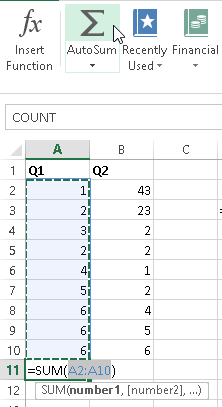## Common Sum Errors

#VALUE! – you have non-integers in the sum formula (not an issue in Excel 2013+)

#REF! – previously referenced column(s) or row(s) does not exist anymore

#NAME? – check formula spelling